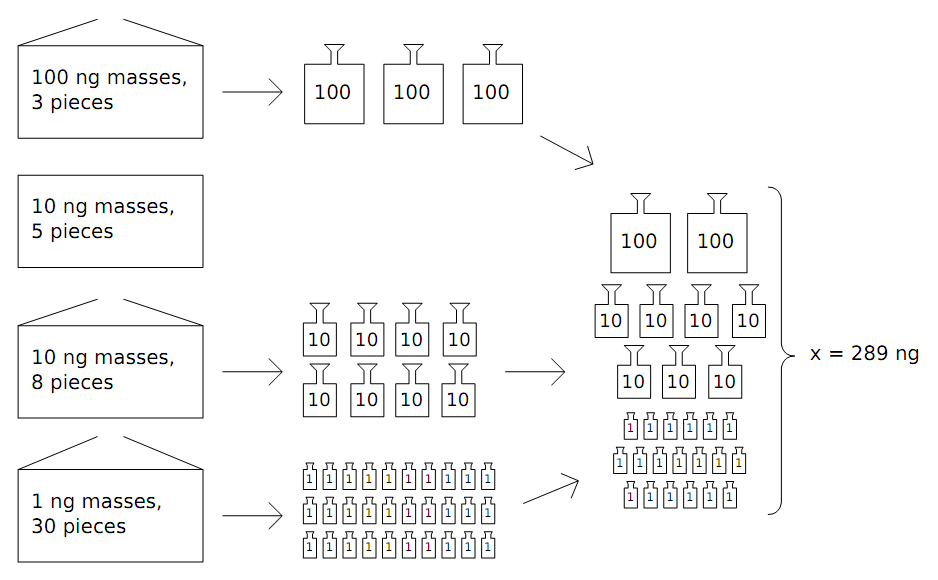Имя: Пароль: Зарегистрироваться Восстановить пароль
24/09/2023 21:41:16

#Рабочее место участника

##Задачи

1889. Exact Measurement

Ограничения: время – 2s/4s, память – 256MiB Ввод: input.txt или стандартный ввод Вывод: output.txt или стандартный выводПослать решение Blockly Посылки Темы Где Обсудить (0)

Peter is working in a secret chemical laboratory. For his new experiment he needs to measure exactly x nanograms of a secret reagent. He has a balance and several standard masses, and his goal is to choose a set of standard masses with total sum equal to x ng.
Standard masses come in n sealed boxes. The i-th box contains qi identical masses of 10^{k_i} ng. Peter wants to open the minimal number of boxes to take a set of masses with the sum of their weights of x ng.Input
The first line of the input file contains two integer numbers x and n (1\ ≤\ x\ ≤\ 10^18, 1\ ≤\ n\ ≤\ 10^5). The next n lines contain pairs of numbers k_i and q_i (0\ ≤\ k_i\ ≤\ 18, 1\ ≤\ q_i\ *\ 10^{k_i}\ ≤\ 10^18)
Output
On the first line output the minimal number of boxes that should be opened. On the second line output the numbers of these boxes in any order. Boxes are numbered in the order they appear in the input file starting from 1. If it is impossible to measure exactly x ng, output a single line with -1.

Sample Input #1

289 4
2 3
1 5
1 8
0 30


Sample Output #1

3
1 3 4


Sample Input #2

300 4
2 3
1 5
1 7
0 30


Sample Output #2

1
1


Sample Input #3

201 1
2 3


Sample Output #3

-1

Source: ACM ICPC NEERC, 2012